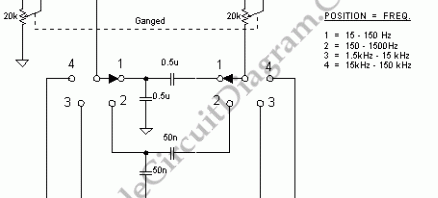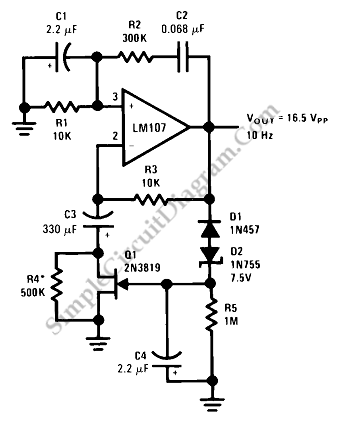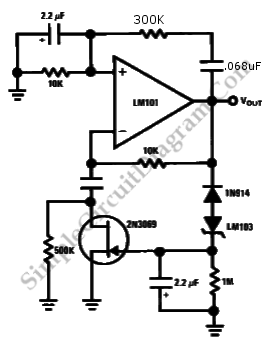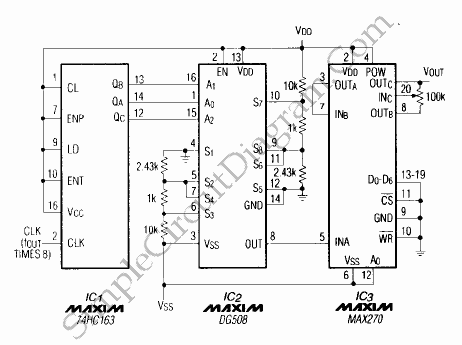## Low Distortion Wien Bridge OscillatorThis Wien bridge oscillator uses incandescent lamp to stabilize its amplitude. This amplitude stabilization gives a low distortion output.  Multiple frequency range selector gives flexible and fine adjustment for the frequency. Here is the schematic diagram of the circuit: The 20k potentiometers is a dual channel pot, which is commonly used in stereo audio circuits. You can many types of […]

## Sine Wave OscillatorThis is a Sine Wave Oscillator circuit. This circuit as known as  amplitude-stabilized sine-wave oscillator. It can gives high purity sine-wave output down to low frequencies with minimum circuit complexity. This circuit has a several advantages such as it does not use the traditional tungsten filament lamp amplitude regulator, so the problem problems associated with a lamp such  as linearity […]

## Wien Bridge Sine Wave OscillatorGetting the right amplifier loop gain is the major problem in producing a low distortion constant amplitude sine wave. However, we can solve this problem with Wien bridge sine wave oscillator. Here is the circuit : This circuit uses the 2N3069 JFET as a voltage variable resistor in the amplifier feedback loop. The voltage reference for the peak sine wave […]